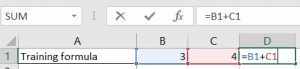# How to use a speadsheet

### How to Use a Spreadsheet

By Dr. Terry KibiloskiAt some point, you may wish to use your computer to do budgets, projections, and other tasks to help you manage your money. Also, since this is tax season, you may wish to use your computer to help you total up all your expenses. One way of using your computer to do these tasks is to use an electronic spreadsheet. So, in this article we look at how to use an electronic spreadsheet.

Microsoft Excel is a popular spreadsheet program.  The information in this article will apply to Microsoft Excel, or to any other computerized spreadsheets.  The computerized spreadsheet is simply a program to replace a manual spreadsheet as shown below.

 Trip Expense 1-Days 5-Days Food 30 150 Gas 20 100 Motel 55 275 Total Expenses 105 525

Look at this manual spreadsheet. Forget it has anything to do with a computer. Does it make sense to you? Could you do the math using your pocket calculator or a stubby pencil? If you were doing everything with a pencil, you would write in the total amount of money you plan on spending each day for each expense. You would then add your expenses in rows 2 through 4 to figure out your total cost per day. To figure the cost of a 5-day trip, you would multiply the cost per day times 5. If you change your mind about the number of days, or the cost of a motel for each day, you have to erase many of your numbers and calculate them all over again. On the other hand, if you use an electronic spreadsheet, it would recalculate everything for you. Let’s see how you would set it up to do this.

Look at the following spreadsheet example.

 A B C 1 Expense 1-day 5-days 2 Food 30 150 3 Gas 20 100 4 Motel 55 275 5 Total Expenses 105 525

Notice that the columns are labeled on the very top as A through C, and the rows are labeled to the very left as 1 through 5. Each cell in an electronic spreadsheet has its own name. In our example, the word motel is in cell A4, and the label 5-days is in cell C1. Although it may appear to be so, we did not type the calculated answers in cells B5 and C2 through C5. Instead, we typed formulas to compute the answers. That way, if we change our mind on the daily cost of an expense, like motel, the formulas will automatically recalculate everything. For example, as soon as you enter a new number in cell B4, the spreadsheet will automatically recalculate the following cells: total expenses in cell B5, motel cost per 5 days in cell C4, and the total expenses in cell C5.

All formulas in an electronic spreadsheet must begin with an equal sign [=]. Math symbols used by electronic spreadsheets are + (add), – (subtract), * (multiply), and / (divide). On a standard computer keyboard, you will find these symbols arranged together on your number keypad. In addition to standard formulas, electronic spreadsheets have special functions that act as shortcuts. The function we use in our example below is the SUM function. You tell the SUM function which cells to add by enclosing in parenthesis the first cell and the last cell in the series, divided by a colon [:]. In cell B5 we are telling the electronic spreadsheet to give us the sum of cells B2 through B4.

 A B C 1 Expense 1-day 5-days 2 Food 30 =C3*5 3 Gas 20 =C4*5 4 Motel 55 =C5*5 5 Total Expenses =SUM(B2:B4) =C2+C3+C4

In cell C5, we are calculating the sum of the number cells in column C by using a simple addition formula: =C2+C3+C4. We could have used the SUM function here if we chose to do so [=SUM(C2:C4)], but we wanted to show you how you can use a simple addition formula to achieve the same result.

At the top of the Microsoft Excel spreadsheet, you will see the formula that is in your selected cell, while on the  spreadsheet itself you will see the number that the formula has calculated.  If you double click a cell that is calculated by a formula, you will also be able to quickly see the formula, and you can edit it within the cell, as well as at the top of the page.In addition to the SUM function, there are many other functions that may be useful when using the electronic spreadsheet. A few functions that are set up identical to the SUM function are the minimum function (MIN), maximum function (MAX) and the average (AVERAGE) function. If we used them on our 1-day column, we would use the following formulas:

 =MIN(B2:B4) =MAX(B2:B4) =AVERAGE(B2:B4)

The MIN function formula would show us the number 20 since that is the smallest (minimum) number in cells B2 through B4. The MAX function formula would show us the number 55 since that is the largest (maximum) number in cells B2 through B4. The AVERAGE function would show us the number 35 since that is the average of the numbers in cells B2 through B4. Notice we had to spell out AVERAGE but only had to use the first three letters of MIN and MAX. That is simply a requirement of Microsoft Excel.

We have only scratched the surface of how to use a spreadsheet.  If you can figure a calculation on paper, you can direct an electronic spreadsheet to do it for you. Spreadsheets are useful for budgets, stocks, vacation planning, etc. Go ahead. Try it. You will be amazed how fast you will get results.

Till next time . . . Happy Computing! J